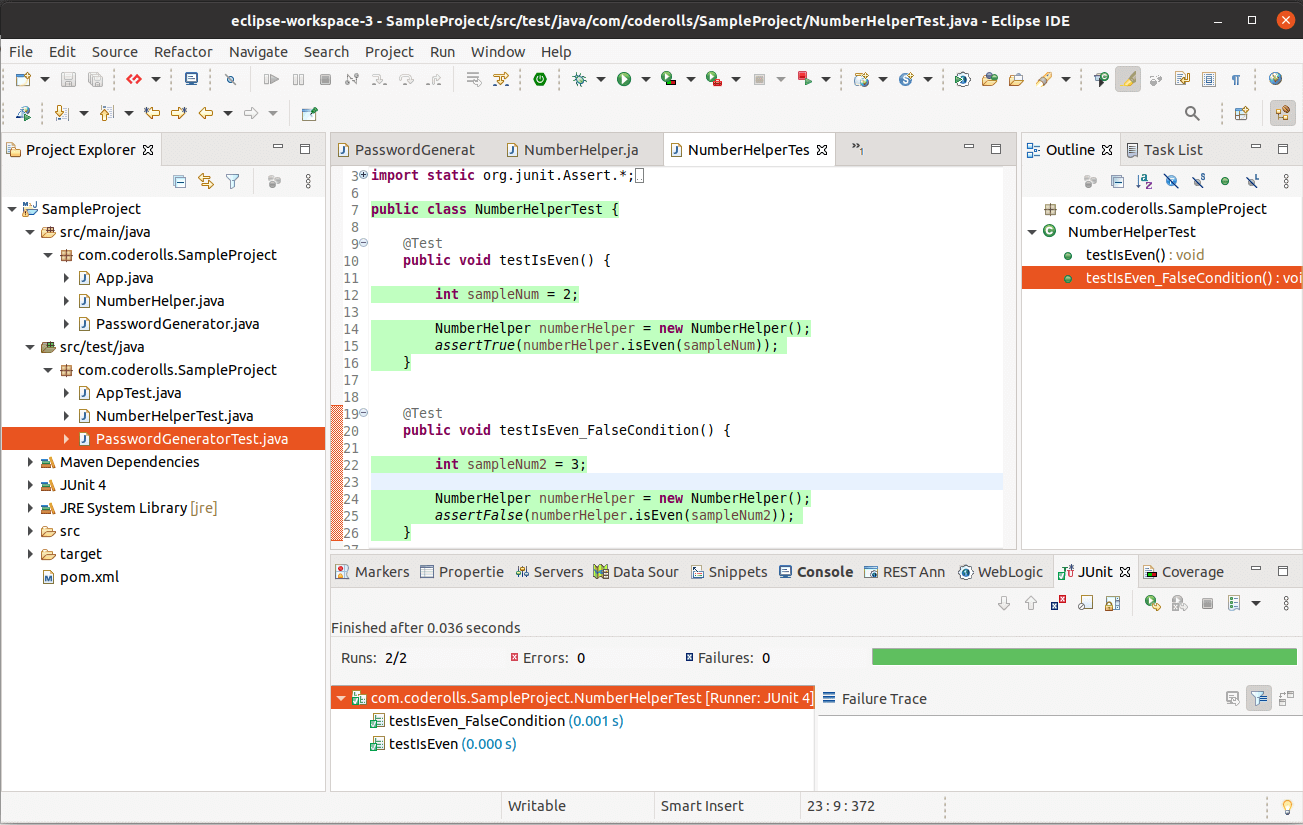Gaurav Kukade Hi 👋🏼 I'm Gaurav Kukade, a software developer. I write tutorials for Java programming language and related technologies.

# assertTrue() and assertFalse() in JUnit With Example

In this tutorial, we will see the `assertTrue()` and `assertFalse()` assertion methods from the JUnit with various examples.

The `Assertions` is a collection of utility methods that support asserting conditions in tests.

## assertTrue(boolean condition)

`assertTrue(boolean condition)` asserts that the supplied `condition` is `true`.

Example:

Here we will take a sample class `NumberHelper.java`. It has `isEven()` which checks if the number is even or not.

The code for `NumberHelper.java` is given below.

``````1
2
3
4
5
6
7
8
9
10
11
12
package com.coderolls.SampleProject;

public class NumberHelper {

public boolean isEven(int num) {

if(num%2==0) {
return true;
}
return false;
}
}
``````

To test the `isEven()` method of `NumberHelper.java` class we will create `NumberHelperTest.java` under `src\test\java` in our maven project.

In `NumberHelperTest.java` we will create a test method `testIsEven()` method to test the `isEven()` method.

``````1
2
3
4
5
6
7
8
9
10
11
12
13
14
15
16
17
18
package com.coderolls.SampleProject;

import static org.junit.Assert.*;

import org.junit.Test;

public class NumberHelperTest {

@Test
public void testIsEven() {

int sampleNum = 2;

NumberHelper numberHelper = new NumberHelper();
assertTrue(numberHelper.isEven(sampleNum));
}

}
``````

As shown in the code, I have taken a sample even number 2.

We know that 2 is an even number so the `isEven()` method must return a `true` value. That’s why we are using `assertTrue()` to assert the behavior of `isEven()` method.

Once you run it as JUnit test case (right-click in the unit test editor, Run as > JUnit Test) you can see the test is passed.

## assertFalse(boolean condition)

`assertFalse(boolean condition)` asserts that the supplied `condition` is `false`.

Example:

We will take the same class and method (`NumberHelper.java` and `isEven()` method) for `assertFalse(boolean condition)` example.

We will add an addition test method in the `NumberHelperTest.java` as `testIsEven_FalseCondition()`.

Here we will take a `sampleNum` as `3`.

We know 3 is not an even number, so `isEven()` method must return `false`. To check it we can use `assertFalse()`.

``````1
2
3
4
5
6
7
8
@Test
public void testIsEven_FalseCondition() {

int sampleNum2 = 3;

NumberHelper numberHelper = new NumberHelper();
assertFalse(numberHelper.isEven(sampleNum2));
}
``````

Once you run this test, it will pass.

I have attached a screenshot below. It shows the test code with the `assertTrue()` and `assertFalse()` test method as well as both the unit test has passed.The example java programs used in the above article can be found at this GitHub repository, coderolls/junit-tutorial.